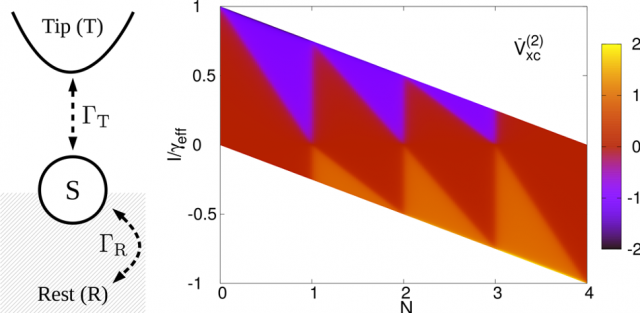# How to extract the interacting spectral function from a ground state DFT calculation

The electronic wave function of an n-electron molecule depends on 3n spatial and n spin coordinates. In a sense, the wave function of a many-electron molecule contains more information than is needed and is lacking in direct physical significance. This has prompted the search for methods that involve fewer variables than the wave function and that can be used to calculate the energy and other properties.

One of these methods is density functional theory (DFT), a theoretical treatment of molecules in which the electron density is considered rather than the wave functions of individual electrons. In other words, a way of describing many-electron, in general, many-fermion, systems in which the energy is a funtional of the density of electrons (fermions).

DFT is without doubt one of the most popular and successful approches for the description of matter with important applications in condensed matter physics, material science and computational chemistry. DFT owes its success to its relative simplicity and low computational cost as compared to other approaches for solving the quantum many-body problem. Despite its simplicity, DFT is in principle exact, that is, it can provide the exact ground state energy and density of many-electron systems. In practice, of course, an approximation for the exchange correlation energy functional is needed and a plethora of such approximations have been suggested.

The spectral function in physics tells you the probability that a particle with a certain momentum has a specific energy. In other words, due to the Heisenberg uncertainty relation, a particle can have an energy that is distributed around a mean. The spectral function describes exactly this distribution and encode information about the (quasi-particle) excitations of the system (energies and lifetimes).

Spectral functions to a large degree determine the transport properties of a many-electron system and can (approximately) be measured, for example, with scanning tunneling microscope (STM) spectroscopy or angular-resolved photoemisson spectroscopy (ARPES). In any case, spectral functions have so far been out of reach for DFT.

Now, Ikerbasque’s researchers David Jacob (UPV/EHU) and Stefan Kurth (UPV/EHU & DIPC) describe 1 a scheme to extract the interacting spectral function (and thus an excited state property) essentially from a ground state DFT calculation. In order to do so, they make use of a recently proposed DFT framework for nonequilibrium steady state transport, the so-called i-DFT approach.

Under certain, well-defined conditions, the i-DFT self-consistent equations for density and current decouple. While the one for the density becomes equivalent to the usual ground-state DFT self-consistency condition, the extra equation for the current can be used to extract the spectral function.Figure 1. (Left) STM-like theoretical setup for measuring the true many-body spectral function of a system in a density functional theory framework. The tip (T), couples very weakly to the sample (S), which in turn is strongly coupled to the rest of the system (R). (Right) Exchange correlation bias

The basic idea is to “measure” the spectral function of a system by means of an STM like setup where a small portion of the system is probed by an STM tip, as shown in the left panel of Figure 1. The tip couples only very weakly to the sample and thus does not influence the system in an essential way. In the ideal STM limit of vanishing coupling to the tip, the system is restored to quasi-equilibrium and the normalized differential conductance yields the exact equilibrium many-body spectral function.

The central idea of i-DFT is that the pair of “densities” – to wit, particle density and the steady current – of the interacting system can in principle exactly be reproduced by an effective system of noninteracting electrons. Calculating normalized differential conductance from i-DFT, the researchers derive an exact relation expressing the interacting spectral function.

Thus, they propose a computationally efficient DFT scheme to calculate the spectral function of an interacting many-electron system. Conceptually, this scheme allows to express the spectral function in terms of the ground state density.

Author: César Tomé López is a science writer and the editor of Mapping Ignorance.

## References

1. David Jacob & Stefan Kurth (2018) Many-Body Spectral Functions from Steady State Density Functional Theory Nano Letters doi: 10.1021/acs.nanolett.8b00255